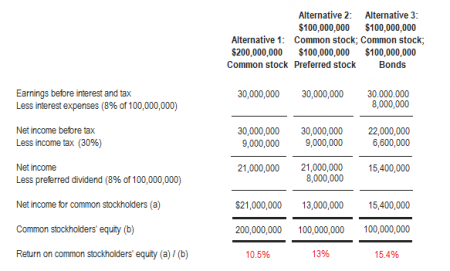# Financial Leverage: Theory and Mathematical Explanation

Financial leverage (or simply leverage) means acquiring assets while using funds provided by means of creditors and desired stockholders for the main benefit of common stockholders. Financial leverage can be a two-edged sword. It may be positive or bad. The following paragraphs explain what on earth is positive and bad financial leverage.

Positive financial leverage:

A positive financial leverage means that the assets acquired with the funds provided by creditors and preferred stockholders generate a rate of return that is higher than the rate of interest or dividend payable to the providers of funds. Positive financial leverage is beneficial for common stockholders. For example, XYZ company obtains a long term debt at a rate of 12%. The company can use the funds to earn an after-tax rate of 14%. The interest on debt is tax deductible. If the tax rate is 40%, the after-tax interest rate would be 7.2% [12% × (1 – 0.4)]. The difference of 6.8% (14% – 7.2%) is, therefore, the benefit of common stockholders.

In this situation, the financial leverage is positive because the after-tax rate of return is higher than the after-tax rate of interest on long-term debts.

Negative financial leverage:

A negative financial leverage occurs when the assets acquired with the debts and preferred stock generate a rate of return that is less than the rate of interest or dividend payable to the providers of debts or preferred stock. Negative financial leverage is a loss for common stockholders.

Impact of income tax on financial leverage:

Debt is considered a more effective source of positive financial leverage than preferred stock because the interest on debt is tax deductible but dividend on preferred stock is not. For explanation of this point, consider the following example.

Example:

Suppose a company is considering to expand its production plants. The expansion can increase the net income before interest and tax by \$30 million and requires a financing of \$200 million. The three proposed ways of financing are as follows:

• By issuing common stock.
• By issuing common stock and 8% preferred stock in the ratio of 1 : 1.
• By issuing common stock and 8% bonds in the ratio of 1 : 1.

The following computations show how obtaining debt can be a more efficient way of generating positive financial leverage than issuing preferred stock.The alternatives in above example generate different return on common stockholders’ equity. The reason of the difference is explained below:

Alternative-1 generates 10.5% return on common stockholders’ equity, there is no debt or preferred stock involved, the leverage is therefore zero.

Alternative-2 generates 13% return on common stockholders’ equity, the preferred stock is present but there is no debt.

Alternative-3 generates 15.4% return on common stockholders’ equity which is the highest of three alternatives. The reason is that the preferred stock is replaced with the debt. The interest on debt is tax deductible while the dividend on preferred stock is not.